Question

# In a random sample of six microwave? ovens, the mean repair cost was ?\$90.00 and the...

In a random sample of six

microwave? ovens, the mean repair cost was ?\$90.00

and the standard deviation was ?\$13.00

Assume the variable is normally distributed and use a? t-distribution to construct a 90?% confidence interval for the population mean ?.

What is the margin of error of ???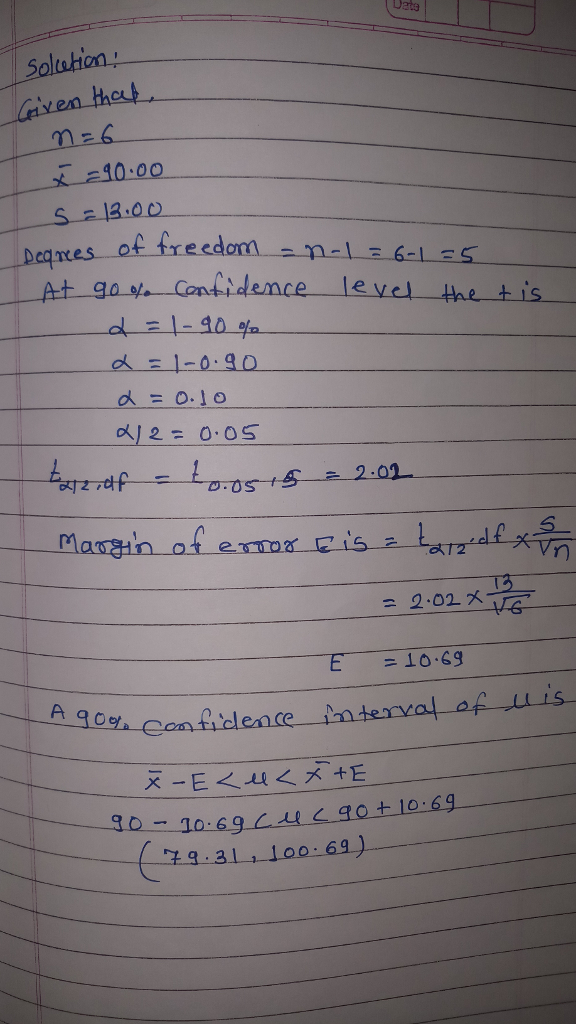#### Earn Coins

Coins can be redeemed for fabulous gifts.

Similar Homework Help Questions
• ### In a random sample of six microwave​ ovens, the mean repair cost was ​\$65.00 and the...

In a random sample of six microwave​ ovens, the mean repair cost was ​\$65.00 and the standard deviation was ​\$12.50. Assume the population is normally distributed and use a​ t-distribution to construct a 90​% confidence interval for the population mean mu. What is the margin of error of mu​? Interpret the results. The 90​% confidence interval for the population mean mu is ​( nothing​, nothing​). ​(Round to two decimal places as​ needed.) The margin of error is nothing. ​(Round to...

• ### In a random sample of fivefive microwave​ ovens, the mean repair cost was ​\$75.0075.00 and the...

In a random sample of fivefive microwave​ ovens, the mean repair cost was ​\$75.0075.00 and the standard deviation was ​\$14.0014.00. Assume the population is normally distributed and use a​ t-distribution to construct a 9090​% confidence interval for the population mean muμ. What is the margin of error of muμ​? Interpret the results.

• ### in a random sample of four microwave ovens, the mean repair cost was 65.00 and the...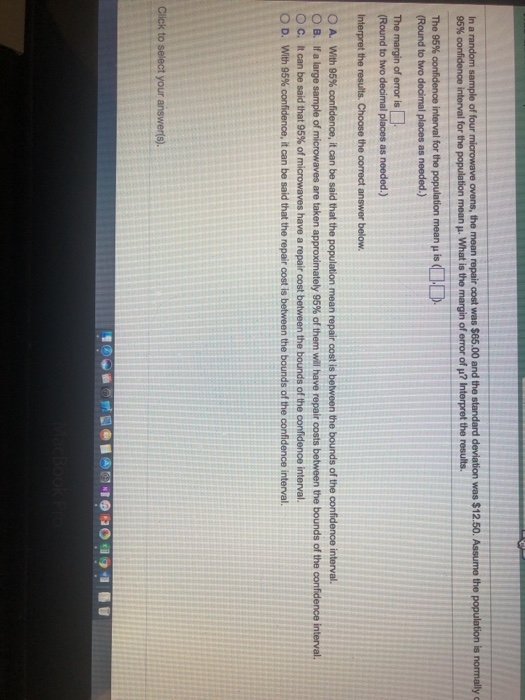in a random sample of four microwave ovens, the mean repair cost was 65.00 and the standard deviation was 12.50 assume the population is normally distributed and use a t-distribution to construct a 95% confidence interval for the population mean u what is the margin of error of u? interpret the results In a Round to two decimal places as needed.)

• ### 6.2.19-T Question Help In a random sample of four microwave ovens, the mean repair cost was...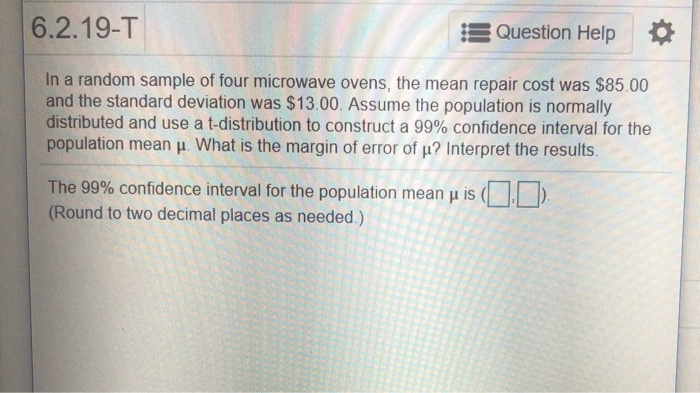6.2.19-T Question Help In a random sample of four microwave ovens, the mean repair cost was \$85.00 and the standard deviation was \$13.00. Assume the population is normally distributed and use a t-distribution to construct a 99% confidence interval for the population mean μ. What is the margin of error of μ? Interpret the results. The 99% confidence interval for the population mean μ is (DD (Round to two decimal places as needed.) 6.2.21-T Question Help In a random sample...

• ### Question Help In a random sample of live microwave ovens, the mean repair cost was \$60.00...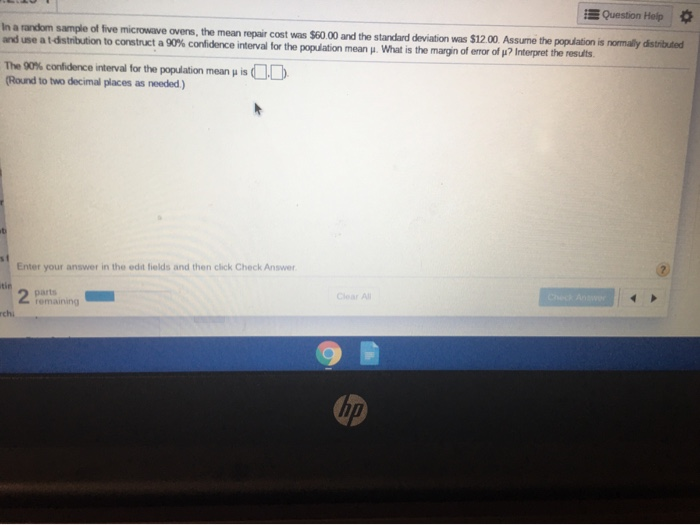Question Help In a random sample of live microwave ovens, the mean repair cost was \$60.00 and the standard deviation was \$12.00. Assume the population is normally distributed and use at distribution to construct a 90% confidence interval for the population mean . What is the margin of error of ? Interpret the results The 90% confidence interval for the population mean (Round to two decimal places as needed.) is C D Enter your answer in the edit fields and...

• ### 6.2.20-T Question Help In a random sample of four mobile devices, the mean repair cost was...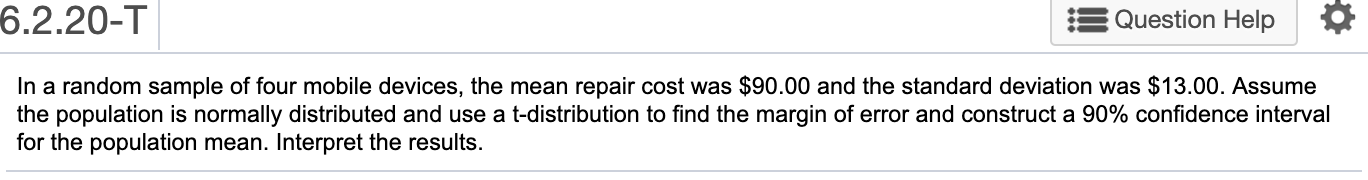6.2.20-T Question Help In a random sample of four mobile devices, the mean repair cost was \$90.00 and the standard deviation was \$13.00. Assume the population is normally distributed and use a t-distribution to find the margin of error and construct a 90% confidence interval for the population mean. Interpret the results.

• ### In a random sample of six mobile​ devices, the mean repair cost was ​\$80.00 and the...

In a random sample of six mobile​ devices, the mean repair cost was ​\$80.00 and the standard deviation was ​\$12.00. Assume the population is normally distributed and use a​ t-distribution to find the margin of error and construct a 95​% confidence interval for the population mean. Interpret the results. The 95​% confidence interval for the population mean mu is

• ### In a random sample of six mobile devices, the mean repair cost was \$70.00 and the...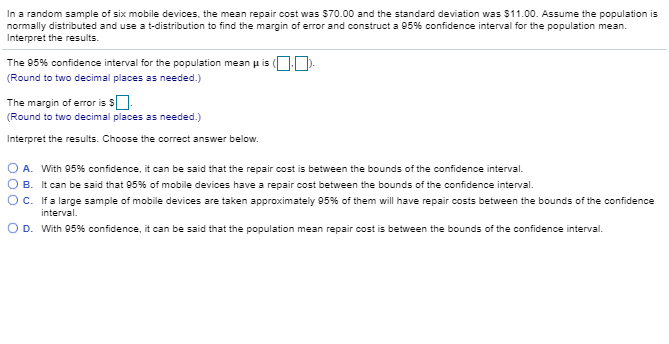In a random sample of six mobile devices, the mean repair cost was \$70.00 and the standard deviation was \$11.00. Assume the population is normally distributed and use a t-distribution to find the margin of error and construct a 95% confidence interval forte population mean. Interpret the results. The 95% confidence interval for the population m ean μ is (DO). Round to two decimal places as needed.) The margin of error is s (Round to two decimal places as needed.)...

• ### in a random sample of six microwave ovens, the mean repair cost was \$60.00 and the...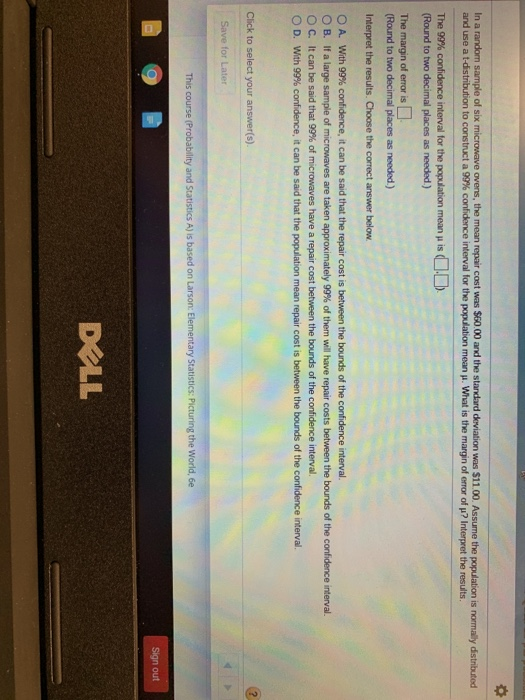in a random sample of six microwave ovens, the mean repair cost was \$60.00 and the standard deviation was \$11.00 Round to two decimal places as needed) (Round to two decimal places as needed.) C. It can be said that 99% of microwaves have a repair cost between the bounds of the c interval DOLL

• ### In a random sample of four mobile devices, the mean repair cost was \$60.00 and the...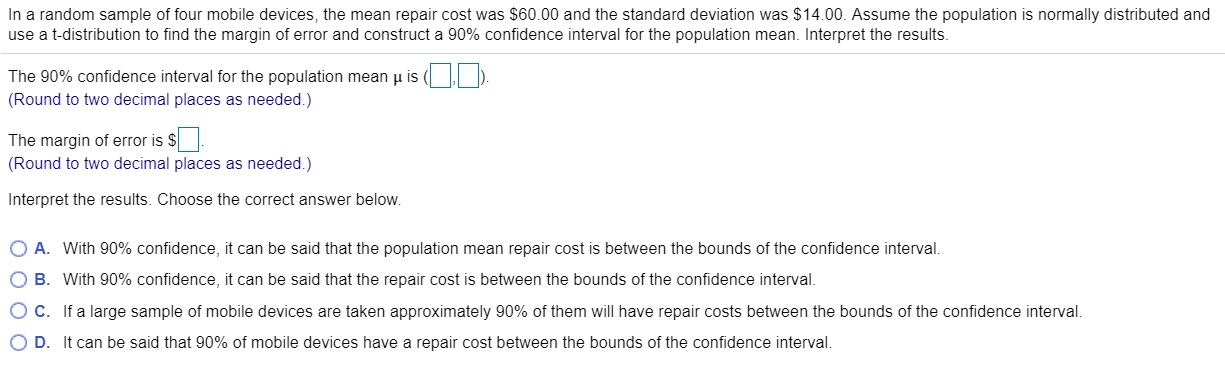In a random sample of four mobile devices, the mean repair cost was \$60.00 and the standard deviation was \$14.00. Assume the population is normally distributed and use a t-distribution to find the margin of error and construct a 90% confidence interval for the population mean. Interpret the results. The 90% confidence interval for the population mean is (DO (Round to two decimal places as needed.) The margin of error is \$ (Round to two decimal places as needed.) Interpret...